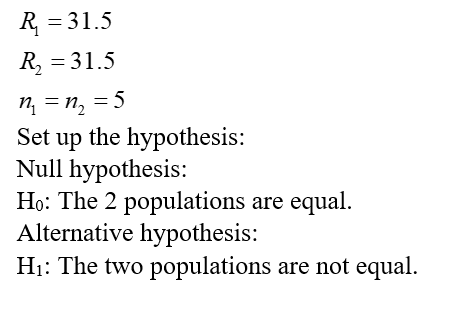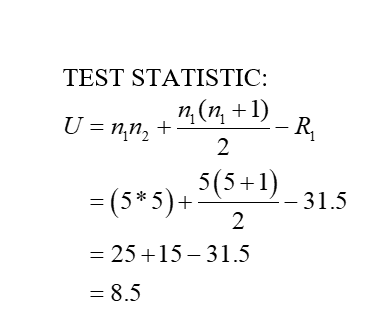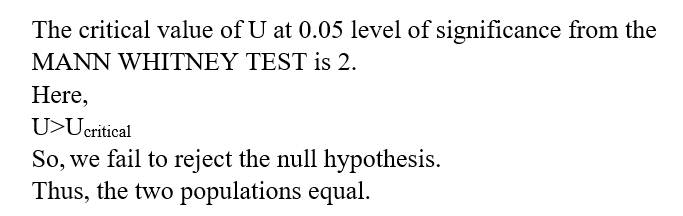# 2. A new chemotherapy drug is released to treat leukemia and researchers suspect that the drug may have fewer side effects than the most commonly used drug to treat leukemia. The two drugs have equivalent efficacy. In order to determine if a larger study should be conducted to look into the prevalence of side effects for the two drugs, set up a Mann-Whitney U test at the alpha equals .05 level and interpret its results.Number of Reported Side-EffectsOld Drug 0 1 3 3 5New Drug 0 0 1 2 4A) We fail to reject H0, which states the two populations are equal at the alpha equals .05 level because the calculated U value of 16.5 is greater than the critical U value of 2.B) We fail to reject H0, which states the two populations are equal at the alpha equals .05 level because the calculated U value of 8.5 is greater than the critical U value of 2.C) We reject H0 in favor of H1, which states the two populations are not equal at the alpha equals .05 level because the calculated U value of 16.5 is greater than the critical U value of 2.D) We reject H0 in favor of H1, which states the two populations are not equal at the alpha equals .05 level because the calculated U value of 8.5 is greater than the critical U value of 2.

Question
35 views

2. A new chemotherapy drug is released to treat leukemia and researchers suspect that the drug may have fewer side effects than the most commonly used drug to treat leukemia. The two drugs have equivalent efficacy. In order to determine if a larger study should be conducted to look into the prevalence of side effects for the two drugs, set up a Mann-Whitney U test at the alpha equals .05 level and interpret its results.
Number of Reported Side-Effects
Old Drug 0 1 3 3 5
New Drug 0 0 1 2 4

A) We fail to reject H0, which states the two populations are equal at the alpha equals .05 level because the calculated U value of 16.5 is greater than the critical U value of 2.
B) We fail to reject H0, which states the two populations are equal at the alpha equals .05 level because the calculated U value of 8.5 is greater than the critical U value of 2.
C) We reject H0 in favor of H1, which states the two populations are not equal at the alpha equals .05 level because the calculated U value of 16.5 is greater than the critical U value of 2.
D) We reject H0 in favor of H1, which states the two populations are not equal at the alpha equals .05 level because the calculated U value of 8.5 is greater than the critical U value of 2.

check_circle

Step 1

Given that,Step 2Step 3...

### Want to see the full answer?

See Solution

#### Want to see this answer and more?

Solutions are written by subject experts who are available 24/7. Questions are typically answered within 1 hour.*

See Solution
*Response times may vary by subject and question.
Tagged in